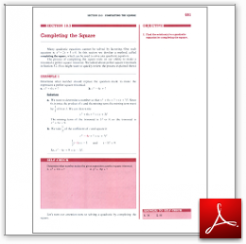Algebra Math Book - Introductory Algebra Thank you for your continual support.

 The power of mathematics rests in the logic of thinking.ID: Sec10-3
Description: Solving a Quadratic Equation by Completing the Square
Price: 1.99

# Algebra Math book - Introductory Algebra - Chapter 10 - Section 3 - Completing the Square

## Section 10.3 - Objectives

3.  Find the solution(s) to a quadratic equation by completing the square.

Navigate to
Previous Section or Next Section
or Chapter 10 Details or Other Chapters

This section of my algebra math book, Introductory Algebra, also includes in the download:

• Cover Sheet